Technical Information Site of Power Supply Design

2021.09.15 Si Power Device

# Basic Operation of PSFB Circuits

Improving the Power Conversion Efficiency of Phase Shift Full Bridge Circuits

ZVS operation in a PSFB circuit is achieved by turning on a MOSFET switch when the output capacitor COSS of the MOSFET is fully discharged and a forward current is flowing through its body diode.

Below are waveforms of the Q1 to Q4 drain currents and the current flowing in the primary-side transformer in a PSFB circuit.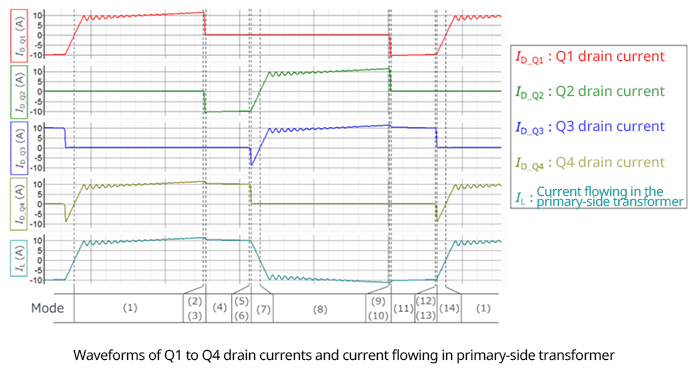Taking the positive current direction to be the direction from drain to source, we see that for each of Q1 to Q4, there is an interval in which a drain current is flowing in the negative direction, that is, an interval in which a forward current is flowing through the body diode of the MOSFET. For example, in the case of Q3, this is the section of Mode (7). During this interval the drain voltage is nearly zero, and so by turning on the MOSFET during this interval, ZVS operation is achieved.

We also see that it is not the case that the same current waveforms, only shifted in phase, occur in the leading leg and the lagging leg. The reasons for the different waveforms can be understood upon considering the current paths for each of the Modes (1) to (14), indicated below the current waveforms. The numbers and positions of the modes are the same as in the timing chart presented previously.

Below, operation and current paths for Modes (1) to (14) are described.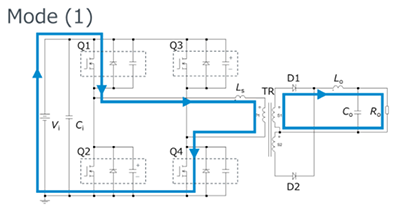・Q1 and Q4 are on, Q2 and Q3 are off. ・With Q2 and Q3 off, the Q2 output capacitor COSS_Q2 and Q3 output capacitor COSS_Q3 are charged. ・Vi is applied to the primary-side transformer. ・Current is flowing in LS, so energy is stored.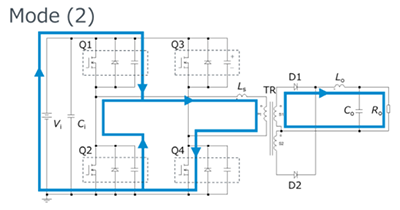・Q1 turns off. ・Since Q1 is off, output capacitor COSS_Q1 is charged. Hence the potential at the Q2 drain falls, and so at the same time discharge of COSS_Q2 begins.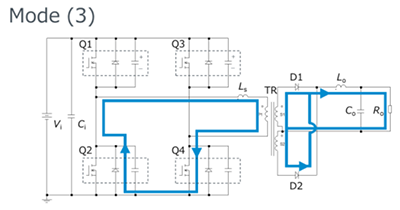・Even after charging of COSS_Q1 and discharging of COSS_Q2 are complete, if energy is stored in LS, current flows through the Q2 body diode （DQ2）, starting freewheeling operation. ・Due to freewheeling operation, energy is not supplied to the secondary side, but because of LO, current continues to flow on the secondary side. The current due to LO is in the forward direction for D1 and D2, so current continues to flow through both diodes.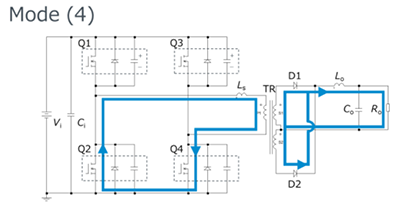・Q2 turns on. At this time DQ2 is conducting, so the Q2 drain-source voltage VDS_Q2 is essentially zero. That is, ZVS operation is achieved, and so a turn-on loss does not occur.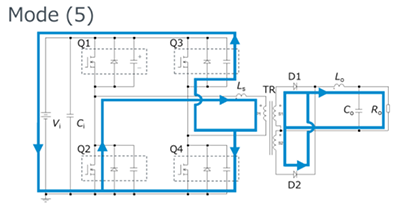・Q4 turns off. ・Due to turn-off of Q4, the Q4 output capacitor COSS_Q4 is charged. At the same time the source potential of Q3 rises, and so the output capacitor COSS_Q3 discharges.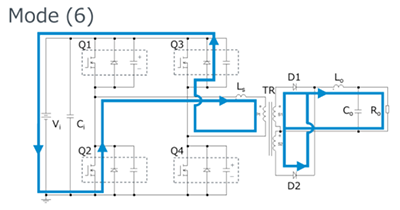・Even after charging of COSS_Q4 and discharging of COSS_Q3 are complete, if energy is stored in LS, current flows through the Q3 body diode （DQ3）, starting freewheeling operation.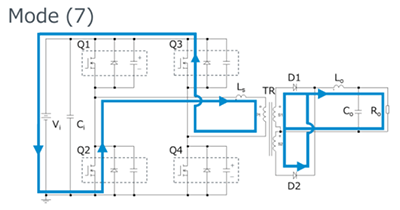・Q3 turns on. At this time DQ3 is conducting, so the Q3 drain-source voltage VDS_Q3 is essentially zero. That is, ZVS operation is achieved, and so a turn-on loss does not occur. ・Because Q3 has turned on, LS rapidly releases energy, and a voltage is applied such that current flows in the direction opposite IL in Modes (1)-(6), so that the current direction is rapidly inverted.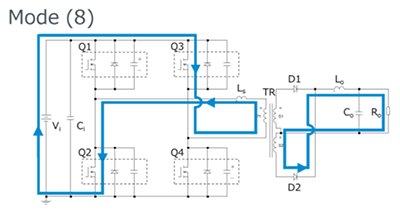・Q2 and Q3 are on, Q1 and Q4 are off. ・Hence COSS_Q1 and COSS_Q4 are charged. ・Vi is applied to the primary-side transformer in the direction opposite that in Mode （1）. ・Current is flowing in LS, so energy is stored.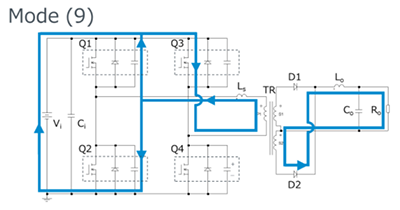・Q2 turns off. ・Because Q2 has turned off, COSS_Q2 is charged, and at the same time COSS_Q1 is discharged.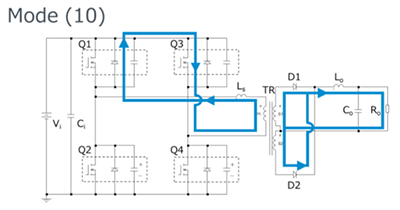・Even after charging of COSS_Q2 and discharging of COSS_Q1 are complete, if energy is stored in LS, current flows through the Q1 body diode （DQ1）, starting freewheeling operation. ・Due to freewheeling operation, energy is not supplied to the secondary side, but because of LO, current continues to flow on the secondary side. The current due to LO is in the forward direction for D1 and D2, so current continues to flow through both diodes.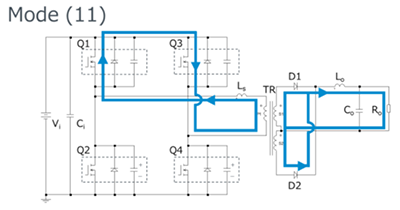・Q1 turns on. At this time DQ1 is conducting, so the Q1 drain-source voltage VDS_Q1 is essentially zero. That is, ZVS operation is achieved, and so a turn-on loss does not occur.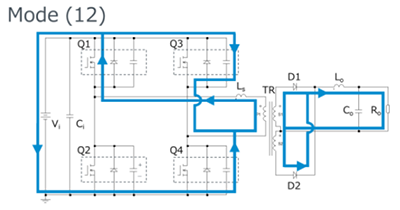・Q3 turns off. ・Because Q3 has turned off, COSS_Q3 is charged, and at the same time COSS_Q4 is discharged.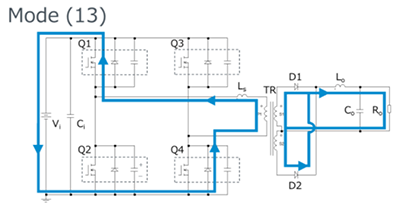・Even after charging of COSS_Q3 and discharging of COSS_Q4 are complete, if energy is stored in LS, current flows through the Q4 body diode （DQ4）, starting freewheeling operation.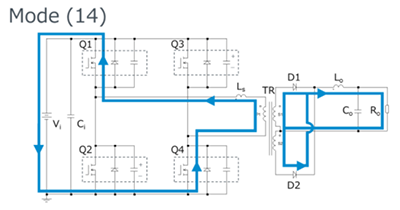・Q4 turns on. At this time DQ4 is conducting, so the Q4 drain-source voltage VDS_Q4 is essentially zero. That is, ZVS operation is achieved, and so a turn-on loss does not occur. ・LS releases energy rapidly, and a voltage is applied such that current flows in the direction opposite IL in Modes (8)-(13), so that the current direction is rapidly inverted.

Due to these changes in current paths, and as explained for Modes (7) and (14) in particular, the turn-on of a MOSFET in the lagging leg causes the input power supply and LS to be series-connected, and the energy in LS decreases rapidly. Because this action does not occur in the leading leg, differences occur in the current waveforms for the leading leg and the lagging leg as a result. Because of these differences in current waveforms, losses are different in the leading-leg MOSFETs and in the lagging-leg MOSFETs, and the amounts of heat generated are different, so care must be exercised in the thermal design.

As in the explanations of Modes (5) and (6) and Modes (12) and (13), in the lagging leg, if the amount of energy stored in LS is not greater than that stored in COSS for a MOSFET, the MOSFET charging and discharging are not completed, and so ZVS operation is not achieved. Hence, if Mode (5) is taken as an example, the condition to achieve ZVS is expressed as equation (1) below. IL1 is IL at the time that Mode (4) ends, and EOSS_Q3 and EOSS_Q4 respectively represent the energy required to complete charging and discharging of the Q3 and Q4 output capacitors.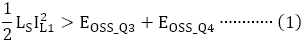From equation (1) we see that under light loading, IL1 is small, so that ZVS operation is not easily achieved, but as the load is increased ZVS operation is more easily realized.

#### Key Points:

・The operation states and current paths, represented as 14 different modes, should be understood.

・Differences in current waveforms occur due to differences in operation. MOSFET losses are different in the leading leg and the lagging leg, and different heat generation results, so that caution must be exercised in thermal design.

・From the equation for the condition for ZVS operation, it is seen that under light loading IL1 is small and so ZVS operation is not readily achieved, but as the load increases, ZVS operation is more easily realized.